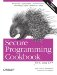# Implementing Fixed-Point Numbers

Problem

You want to perform computations on real numbers using a fixed-point representation of a real number rather than using a floating-point type.

Solution

Example 11-40 provides the implementation of a fixed-point real number, where the number of places to the right of the binary point is a template parameter. For instance basic_fixed_real<10> has 10 binary digits to the right of the binary point, allowing it to represent numbers up to a precision of 1/1,024.

Example 11-40. Representing real numbers using a fixed-point implementation

```#include

using namespace std;

template
struct BasicFixedReal
{
typedef BasicFixedReal self;
static const int factor = 1 << (E - 1);
BasicFixedReal( ) : m(0) { }
BasicFixedReal(double d) : m(static_cast(d * factor)) { }
self& operator+=(const self& x) { m += x.m; return *this; }
self& operator-=(const self& x) { m -= x.m; return *this; }
self& operator*=(const self& x) { m *= x.m; m >>= E; return *this; }
self& operator/=(const self& x) { m /= x.m; m *= factor; return *this; }
self& operator*=(int x) { m *= x; return *this; }
self& operator/=(int x) { m /= x; return *this; }
self operator-( ) { return self(-m); }
double toDouble( ) const { return double(m) / factor; }

// friend functions
friend self operator+(self x, const self& y) { return x += y; }
friend self operator-(self x, const self& y) { return x -= y; }
friend self operator*(self x, const self& y) { return x *= y; }
friend self operator/(self x, const self& y) { return x /= y; }

// comparison operators
friend bool operator==(const self& x, const self& y) { return x.m == y.m; }
friend bool operator!=(const self& x, const self& y) { return x.m != y.m; }
friend bool operator>(const self& x, const self& y) { return x.m > y.m; }
friend bool operator<(const self& x, const self& y) { return x.m < y.m; }
friend bool operator>=(const self& x, const self& y) { return x.m >= y.m; }
friend bool operator<=(const self& x, const self& y) { return x.m <= y.m; }
private:
int m;
};

typedef BasicFixedReal<10> FixedReal;

int main( ) {
FixedReal x(0);
for (int i=0; i < 100; ++i) {
x += FixedReal(0.0625);
}
cout << x.toDouble( ) << endl;
}```

The program in Example 11-40 outputs:

`6.25`

Discussion

A fixed-point number, like a floating-point number, is an approximate representation of a real number. A floating-point number is stored as a mantissa (m), and an exponent (e), to form the equation m * be, where b is some constant.

A fixed-point number is almost the same but the exponent is also a constant. This constant is passed to the basic_fixed_real in Example 11-40 as a template parameter.

By representing e as a constant, it allows fixed-point numbers to be represented internally as integers and for the arithmetic operations on them to be performed using integer artithmetic. This can often improve the speed of basic arithmetic operations especially addition and subtraction.

Fixed-point representations are less flexible than floating-point numbers, as they can only represent a narrow range of values. The fixed_real type in Example 11-40 has a range that can only represent values from -2,097,151 to +2,097,151 with a precision of 1/1,024.

Implementing addition and subtraction of fixed-point numbers is straightforward enough: I simply add or subtract the underlying representation. To perform division and multiplication, I need an extra step of shifting the mantissa left or right to adjust for the binary point.Secure Programming Cookbook for C and C++: Recipes for Cryptography, Authentication, Input Validation & More
ISBN: 0596003943
EAN: 2147483647
Year: 2006
Pages: 241